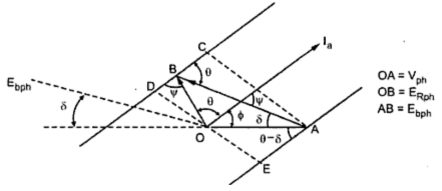### Alternative Expression for Power Developed by a Synchronous Motor

Consider the phasor diagram of a synchronous motor running on leading power factor cosΦ as shown in the Fig. 1.Fig. 1
The line CD is drawn at an angle θ to AB.
The lines AC and DE are perpendicular to CD and AE.
and angle between AB = Ebph and Iaph is also ψ.
The mechanical per phase power developed is given by,
In triangle OBD,
BD = OB cosψ = IZs cosψ
OD = OB sin ψ = Ia  Zs sin
Now    BD = CD - BC = AE - BC
Substituting in (2),
IZs cosψ = Vph  cos (θ-δ) - E cosθ
All values are per phase values
Substituting (3) in (1),
This is the expression for the mechanical power developed interms of the load angle δ and the internal machine angle θ, for constant voltage Vph  and constant Eph  i.e. excitation.